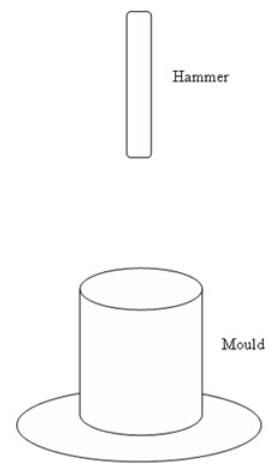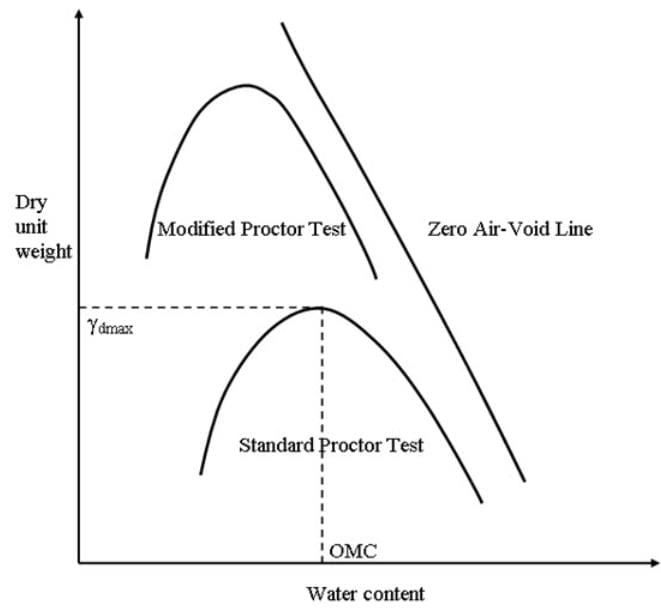Introduction & Laboratory Tests of Compaction, Soil Mechanics

# Introduction & Laboratory Tests of Compaction, Soil Mechanics - Notes | Study Soil Mechanics Notes- Agricultural Engineering - Agricultural Engineering

 1 Crore+ students have signed up on EduRev. Have you?

Introduction
Densification of soils by applying mechanical energy is called compaction. Due to compaction the volume of air voids reduces, density of the soil increases. This is one of the common methods of soil improvement. The compressibility and permeability of the soil decrease due to compaction. Compaction decreases the settlement of soil and increases the bearing capacity of the soil.
Laboratory Tests

The compaction can be done in laboratory by Standard Proctor Test or Modified Proctor Test. In case of Standard Proctor Test, a mould of standard of volume 944 cc is filled with soil in three layers. Each layer is compacted by 25 blows of a hammer of weight 2.495 (or 2.5 kg) kg. The falling height of the hammer is fixed at 304.8 mm (or 30 cm) each time. This process is done for different water content. The compaction mould with hammer is shown in Figure 14.1. The wet unit weight ($\gamma$t) of the soil is calculated each time by knowing the weight of the wet soil and volume of the mould (944 cc). The amount of water content is measured. Once the bulk or wet unit weight and water content (w) of the soil are calculated, the dry unit weight ( $\gamma$ d) of the soil is calculated as:

${\gamma _d}={{{\gamma _t}} \over {1 + w}}$                          (14.1)

The dry unit weight is plotted at different water content. This curve is called as ‘compaction curve’. Figure 14.2 shows a typical compaction curve for clay. The compaction curve has significant importance in case of clayey soil. From the compaction curve, maximum dry unit weight ($\gamma$dmax) and optimum water content (OMC) are obtained (as shown is Figure 14.2). The specification of compaction in the field is decided based on maximum dry unit weight or/and optimum water content. The typical range of maximum dry unit weight of soil is 16 to 20 kN/mwith a wide range of 13 to 24 kN/m3. Similarly, the typical range of OMC is 10 to 20% with a wide range of 5 to 30% (Ranjan and Rao, 2003).

In case of Modified Standard Proctor Test, a mould of standard of volume 944 cc is filled with soil in five layers. Each layer is compacted by 25 blows of a hammer of weight 4.54 kg (or 4.5 kg). The falling height of the hammer is fixed at 457.2 mm (or 45cm) each time. This process is done for different water content.

The Indian standard equivalent, the Standard Proctor Test is called the light compaction test (IS: 2720, Part VII, 1974) and Modified Standard Proctor Test is called the heavy compaction test (IS: 2720, Part VIII, 1983). In case of light compaction test, a mould of standard of volume 1000 cc is filled with soil in three layers. Each layer is compacted by 25 blows of a hammer of weight 2.6 kg. The falling height of the hammer is fixed at 310 mm each time.  In case of heavy compaction test, a mould of standard of volume 1000 cc is filled with soil in five layers. Each layer is compacted by 25 blows of a hammer of weight 4.9 kg. The falling height of the hammer is fixed at 450 mm each time.

The dry unit weight can be written in terms of water content and degree of saturation as:

${\gamma _d}={G \over {1 + e}}{\gamma _w}$

$=\frac{G}{{1+\frac{{wG}}{S}}}{\gamma _w}$                       (14.2)

where G is the specific gravity of the soil, w is the water content, S is the degree of saturation, e is the void ratio and gw is the unit weight of water generally taken as 10 kN/m3. For completely saturated soil, the dry unit weight of the soil is calculated from Eq. (14.2) by putting S =1. The line (unit weight vs water content for a particular specific gravity) corresponding to the 100% degree of saturated is called Zero Air-Void Line (as shown in Figure 14.2). For a particular specific gravity and water content the obtained dry unit weight by putting S = 1 is called Zero Air Void Density or Zero Air Void Unit Weight. The Zero Air Void Unit Weight can be achieved only theoretically, in field it can not be possible to achieve it. What ever compaction energy is applied in the soil, some air will still remain within the soil voids.Fig. 14.1. Compaction mould with hammer.Fig. 14.2. Typical compaction curves of clay.

From the Figure 14.2 it is observed that in case of modified proctor test, the maximum dry unit weight increases as compared to the standard proctor test. However, OMC is more in case of standard proctor test as compared to the modified proctor test. Thus, as the compaction energy increases maximum dry unit weight increases with a decreasing rate.

The document Introduction & Laboratory Tests of Compaction, Soil Mechanics - Notes | Study Soil Mechanics Notes- Agricultural Engineering - Agricultural Engineering is a part of the Agricultural Engineering Course Soil Mechanics Notes- Agricultural Engineering.
All you need of Agricultural Engineering at this link: Agricultural Engineering

## Soil Mechanics Notes- Agricultural Engineering

64 docs
 Use Code STAYHOME200 and get INR 200 additional OFF

## Soil Mechanics Notes- Agricultural Engineering

64 docs

Track your progress, build streaks, highlight & save important lessons and more!

,

,

,

,

,

,

,

,

,

,

,

,

,

,

,

,

,

,

,

,

,

,

,

,

;